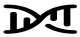# One-sample MR

MR analyses in which the genetic IV-risk factor association and genetic IV-outcome association are from the same sample and individual-level data is used to derive the MR estimate.

Genetic IVs must fulfil the same IV assumptions. The gene-outcome model must be correctly specified, but the gene-exposure model can be miss-specified. One-sample MR is more prone to data overfitting than two-sample MR. Though, two-sample MR may be similar to one-sample if there is overlap of participants in the two samples. In one-sample MR, weak instrument bias will tend to bias towards the confounded multivariable regression estimate.Figure 2.4 - One-sample Mendelian randomization. (A) MR relies on the following three core assumptions: (1) the genetic variant(s) being used as an instrument (Z) is associated with the exposure (X); (2) the instrument in independent of measured and unmeasured confounders (U) of the association between the exposure (X) and outcome (Y); and (3) there is no independent pathway between the instrument (Z) and outcome (Y) other than through the exposure (X) – otherwise known as horizontal pleiotropy or the exclusion restriction assumption. (B) MR can be perceived as being analogous to a randomized controlled trial (RCT), whereby the random assortment of alleles at conception is equivalent to the randomization method with an RCT. This randomization process produces groups of individuals who differ with respect to the intervention (in the case of MR, genetic variation) and between which confounders are equally distributed. Therefore, any differences observed in the outcome of interest between these randomly allocated groups should be due to the exposure with which the genetic variant(s) are associated. (C) In the most simple of scenarios, the causal estimate of the association between the exposure (X) and outcome (Y) can be derived using the instrumental variable ratio method (otherwise known as the Wald ratio), where 𝛽𝐼𝑉 is the causal estimate derived from instrumental variable (i.e., MR) analyses, 𝛽𝑌𝑍 is the association between the instrument (Z) and the outcome (Y) and 𝛽𝑋𝑍 is the association between the instrument (Z) and the exposure (X).